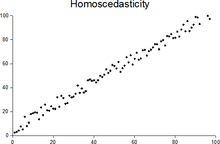# HomoscedasticityPlot with random data showing homoscedasticity.

In statistics, a sequence or a vector of random variables is homoscedastic /ˌhmskəˈdæstɪk/ if all random variables in the sequence or vector have the same finite variance. This is also known as homogeneity of variance. The complementary notion is called heteroscedasticity. The spellings homoskedasticity and heteroskedasticity are also frequently used.

The assumption of homoscedasticity simplifies mathematical and computational treatment. Serious violations in homoscedasticity (assuming a distribution of data is homoscedastic when in reality it is heteroscedastic /ˌhɛtərskəˈdæstɪk/) may result in overestimating the goodness of fit as measured by the Pearson coefficient.

## Assumptions of a regression model

As used in describing simple linear regression analysis, one assumption of the fitted model (to ensure that the least-squares estimators are each a best linear unbiased estimator of the respective population parameters, by the Gauss–Markov theorem) is that the standard deviations of the error terms are constant and do not depend on the x-value. Consequently, each probability distribution for y (response variable) has the same standard deviation regardless of the x-value (predictor). In short, this assumption is homoscedasticity. Homoscedasticity is not required for the estimates to be unbiased, consistent, and asymptotically normal.

## Testing

Residuals can be tested for homoscedasticity using the Breusch–Pagan test, which regresses squared residuals on the independent variables. Since the Breusch–Pagan test is sensitive to departures from normality, the Koenker–Basset or 'generalized Breusch–Pagan' test is used for general purposes. Testing for groupwise heteroscedasticity requires the Goldfeld–Quandt test.

## Homoscedastic distributions

Two or more normal distributions,, are homoscedastic if they share a common covariance (or correlation) matrix,. Homoscedastic distributions are especially useful to derive statistical pattern recognition and machine learning algorithms. One popular example is Fisher's linear discriminant analysis.

The concept of homoscedasticity can be applied to distributions on spheres.